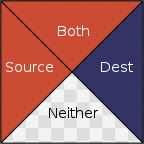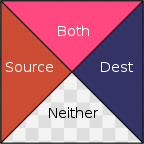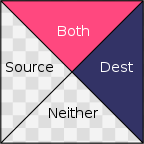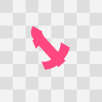# Porter/Duff Compositing and Blend Modes

In the Porter/Duff compositing algebra, images are equipped with an alpha channel that determines on a per-pixel basis whether the image is there or not. When the alpha channel is 1, the image is fully there, when it is 0, the image isn’t there at all, and when it is in between, the image is partially there. In other words, the alpha channel describes the shape of the image, it does not describe opacity. The way to think of images with an alpha channel is as irregularly shaped pieces of cardboard, not as colored glass.

Consider these two images:When we combine them, each pixel of the result can be divided into four regions:One region where only the source is present, one where only the destination is present, one where both are present, and one where neither is present.

By deciding on what happens in each of the four regions, various effects can be generated. For example, if the destination-only region is treated as blank, the source-only region is filled with the source color, and the ‘both’ region is filled with the destination color like this:The effect is as if the destination image is trimmed to match the source image, and then held up in front of it:The Porter/Duff operator that does this is called “Dest Atop”.

There are twelve of these operators, each one characterized by its behavior in the three regions: source, destination and both. The ‘neither’ region is always blank. The source and destination regions can either be blank or filled with the source or destination colors respectively.

The formula for the operators is a linear combination of the contents of the four regions, where the weights are the areas of each region:

$A_\text{src} \cdot [s] + A_\text{dest} \cdot [d] + A_\text{both} \cdot [b]$

Where $[s]$ is either 0 or the color of the source pixel, $[d]$ either 0 or the color of the destination pixel, and $[b]$ is either 0, the color of the source pixel, or the color of the destination pixel. With the alpha channel being interpreted as coverage, the areas are given by these formulas:

$A_\text{src} = \alpha_\text{s} \cdot (1 - \alpha_\text{d})$
$A_\text{dst} = \alpha_\text{d} \cdot (1 - \alpha_\text{s})$
$A_\text{both} = \alpha_\text{s} \cdot \alpha_\text{d}$

The alpha channel of the result is computed in a similar way:

$A_\text{src} \cdot [\text{as}] + A_\text{dest} \cdot [\text{ad}] + A_\text{both} \cdot [\text{ab}]$

where $[\text{as}]$ and $[\text{ad}]$ are either 0 or 1 depending on whether the source and destination regions are present, and where $[\text{ab}]$ is 0 when the ‘both’ region is blank, and 1 otherwise.

Here is a table of all the Porter/Duff operators:

 $[\text{s}]$ $[\text{d}]$ $[\text{b}]$ Src $s$ $0$ s Atop $0$ $d$ s Over $s$ $d$ s In $0$ $0$ s Out $s$ $0$ $0$ Dest $0$ $d$ d DestAtop $s$ $0$ d DestOver $s$ $d$ d DestIn $0$ $0$ d DestOut $0$ $d$ $0$ Clear $0$ $0$ $0$ Xor $s$ $d$ $0$

And here is how they look:Despite being referred to as alpha blending and despite alpha often being used to model opacity, in concept Porter/Duff is not a way to blend the source and destination shapes. It is way to overlay, combine and trim them as if they were pieces of cardboard. The only place where source and destination pixels are actually blended is along the antialiased edges.

Blending
Photoshop and the Gimp have a concept of layers which are images stacked on top of each other. In Porter/Duff, stacking images on top of each other is done with the “Over” operator, which is also what Photoshop/Gimp use by default to composite layers:Conceptually, two pieces of cardboard are held up with one in front of the other. Neither shape is trimmed, and in places where both are present, only the top layer is visible.

A layer in these programs also has an associated Blend Mode which can be used to modify what happens in places where both are visible. For example, the ‘Color Dodge’ blend mode computes a mix of source and destination according to this formula:

$\begin{equation*} B(s,d)= \begin{cases} 0 & \text{if $$d=0$$,} \\ 1 & \text{if $$d \ge (1 - s)$$,} \\ d / (1 - s) & \text{otherwise} \end{cases} \end{equation*}$

The result is this:Unlike with the regular Over operator, in this case there is a substantial chunk of the output where the result is actually a mix of the source and destination.

Layers in Photoshop and Gimp are not tailored to each other (except for layer masks, which we will ignore here), so the compositing of the layer stack is done with the source-only and destination-only region set to source and destination respectively. However, there is nothing in principle stopping us from setting the source-only and destination-only regions to blank, but keeping the blend mode in the ‘both’ region, so that tailoring could be supported alongside blending. For example, we could set the ‘source’ region to blank, the ‘destination’ region to the destination color, and the ‘both’ region to ColorDodge:Here are the four combinations that involve a ColorDodge blend mode:In this model the original twelve Porter/Duff operators can be viewed as the results of three simple blend modes:

 Source: $B(s, d) = s$ Dest: $B(s, d) = d$ Zero: $B(s, d) = 0$

In this generalization of Porter/Duff the blend mode is chosen from a large set of formulas, and each formula gives rise to four new compositing operators characterized by whether the source and destination are blank or contain the corresponding pixel color.

Here is a table of the operators that are generated by various blend modes:The general formula is still an area weighted average:

$A_\text{src} \cdot [s] + A_\text{dest} \cdot [d] + A_\text{both}\cdot B(s, d)$

where [s] and [d] are the source and destination colors respectively or 0, but where $B(s, d)$ is no longer restricted to one of $0$, $s$, and $d$, but can instead be chosen from a large set of formulas.

The output of the alpha channel is the same as before:

$A_\text{src} \cdot [\text{as}] + A_\text{dest} \cdot [\text{ad}] + A_\text{both} \cdot [\text{ab}]$

except that [ab] is now determined by the blend mode. For the Zero blend mode there is no coverage in the both region, so [ab] is 0; for most others, there is full coverage, so [ab] is 1.